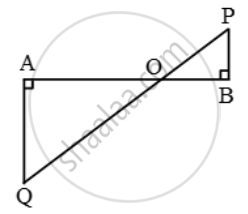Share

# In figure, QA and PB are perpendicular to AB. If AO = 10 cm, BO = 6 cm and PB = 9 cm. Find AQ - CBSE Class 10 - Mathematics

ConceptSimilarity Examples and Solutions

#### Question

In figure, QA and PB are perpendicular to AB. If AO = 10 cm, BO = 6 cm and PB = 9 cm. Find AQ

#### Solution

In triangles AOQ and BOP, we have

∠OAQ = ∠OBP [Each equal to 90º]

∠AOQ = ∠BOP [Vertically opposite angles]

Therefore, by AA-criterion of similarity

∆AOQ ~ ∆BOP\Rightarrow \frac{AO}{BO}=\frac{OQ}{OP}=\frac{AO}{BP}

\Rightarrow \frac{AO}{BO}=\frac{AQ}{BP}\Rightarrow\frac{10}{6}=\frac{AQ}{9}

\Rightarrow AQ=\frac{10\times 9}{6}=15\text{ }cm

Is there an error in this question or solution?

#### Video TutorialsVIEW ALL 

Solution In figure, QA and PB are perpendicular to AB. If AO = 10 cm, BO = 6 cm and PB = 9 cm. Find AQ Concept: Similarity Examples and Solutions.
S